In Part 3, I introduced some aspects of object-oriented programming (OOP) for Kotlin. In this article, I will continue to introduce other aspects of OOP.

## Interface

We can use interfaces the way we are used in Java. A Kotlin interface method can have a default implementation. Unlike Java 8, which requires the  `default ` keyword, Kotlin has no special annotation for such methods: you just provide a method body. Example:

`interface Person {`
`   fun canWork():String = "I can unknown!"`
`}`

Kotlin interfaces can have properties but these need to be abstract or to provide accessor implementations. Example:

`interface Person {`
`      var Name:String //abstract`
`      var Gender:String // abstract`
`      fun canWork():String = "I can unknown!"`
`}`

We can implement interfaces in a class look like this:

`class Student:Person`
`{`
`    override var Name:String=""`
`        get() = field.toUpperCase()`
`        set(value){`
`            field="I am \$value"`
`        }`
`    override var Gender:String =""`
`    var StudentID:String = ""`
`    var University:String=""`
`    override fun canWork(): String {`
`        return "\$Name .I am learning at \$University. My ID is \$StudentID and my gender is \$Gender \n"`
`    }`
`}`

Kotlin doesn’t support multiple inheritances, however, the same thing can be achieved by implementing more than two interfaces at a time.

## Data Classes

Like most other aspects of Kotlin, data classes aim to reduce the amount of boilerplate code you write in your project. Data classes provide compiler-generated  `equals()` , ` hashCode()` ,  `toString()` ,  `copy()` , and other methods. An example of a normal class:

`class Person {`
`        var Name: String = ""`
`}`

We create an object from the  `Person ` class:

`val p = Person()`
`println( p.toString())`

The result can look like this:

` …Person@5281e6c0`

Now, we change the  `Person ` class to become a data class by using  `data ` keyword as follows:

`data class Person(var Name:String = "")`

We create an object from the Person class again:

`val p = Person(Name="Minh")`
`println( p.toString())`

The result can look like this:

`Person(Name=Minh)`

If we declare some properties inside  `Person ` class body as follows:

`data class Person1(var Name:String =""){`
`     var Gender:String=""`
`     var Address:String=""   `
` }`

Creating an object

`val p = Person(Name="Minh")`
`p.Gender ="Male"`
`p.Address="New York"`
`println( p.toString())`

The result also looks like this:

`Person(Name=Minh)`

This is because the compiler only uses the properties defined inside the primary constructor for  `toString()` ,  `equals()` ,  `hashCode()` , and `copy()` implementations.

We also can use the `copy()` method copy an object altering some of its properties. An example:

`val person = Person(Name="Minh", Gender="Male",Address = "NewYork")`
`val otherperson = person.copy(Address = "Chicago")`
`println( p.toString()) // The result: Person(Name=Minh,Gender=Male,Address=Chicago)`

### Nested and Inner Classes

In Kotlin, classes can be nested in other classes:

`class FlyAnimal {`
`    //…..`
`    class Bird {`
`//…`
`    }`
`}`

A class may be marked as  `inner ` to be able to access members of outer class. Inner classes carry a reference to an object of an outer class:

`class FlyAnimal {`
`    private val wings: Int = 2`
`    inner class Bird {`
`        var birdWings = wings`
`    }`
`}`
`println (FlyAnimal().Bird().birdWings.toString()) // 2`

### Sealed Classes

When you evaluate an expression using the  `when ` construct, the Kotlin compiler forces you to check for the default option. Example:

`interface Expr`
`class Num(val value: Int) : Expr`
`class Sum(val left: Expr, val right: Expr) : Expr`
`fun eval(e: Expr): Int =`
`when (e) {`
`is Num -> e.value`
`is Sum -> eval(e.right) + eval(e.left)`
`else -> throw IllegalArgumentException("Unknown expression")`
`}`

If you add a new subclass, the compiler won’t detect that something has changed. If you forget to add a new branch, the default one will be chosen, which can lead to subtle bugs. Kotlin provides a solution to this problem: : sealed classes. Let’s look at the following example:

`sealed class Expr {`
`class Num(val value: Int) : Expr()`
`class Sum(val left: Expr, val right: Expr) : Expr()`
`}`
`fun eval(e: Expr): Int =`
`when (e) {`
`is Expr.Num -> e.value`
`is Expr.Sum -> eval(e.right) + eval(e.left)`
`}`

If you handle all subclasses of a sealed class in a when statement, you don’t need to provide the default branch.

## An Android Application

In this application, I created an UI as follows: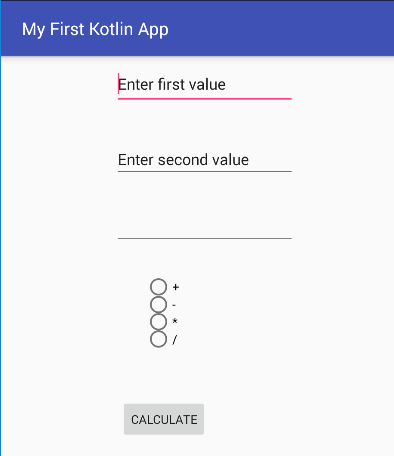We can input the first value, the second value, and choose an operator as follows: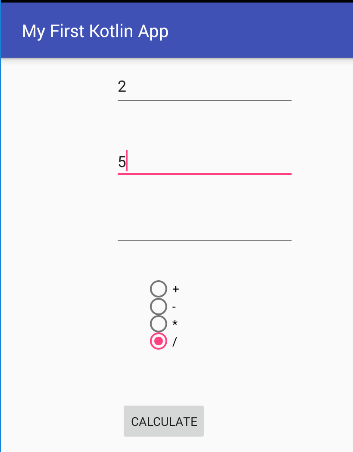Click the  `CALCULATE ` button, the result can look like this: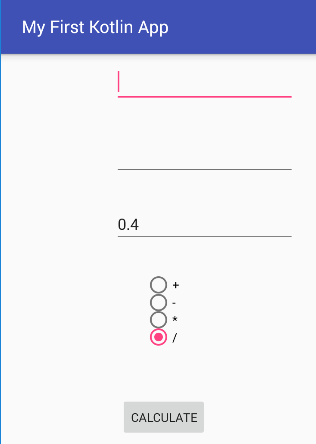Some controls are used in this application:

 Control ID attribute Text attribute EditText value1 @string/value1 EditText value2 @string/value2 EditText result @string/result RadioButton add @string/add RadioButton sub @string/sub RadioButton mul @string/mul RadioButton div @string/div RadioGroup operators Button calculate @string/calculate

(You can see source code of activity_main.xml and strings.xml files here)

In the MainActivity.kt, the first, I created the sealed class named `Expr` and its subclasses:

`sealed class Expr {`
`    class Num(val value: Double) : Expr()`
`    class Add(val left: Expr, val right: Expr) : Expr()`
`    class Sub(val left: Expr, val right: Expr) : Expr()`
`    class Mul(val left: Expr, val right: Expr) : Expr()`
`    class Div(val left: Expr, val right: Expr) : Expr()`
`}`

In MainActivity class, I created the  `eval ` function:

`fun eval(e: Expr): Double =`
`            when (e) {`
`                    is Expr.Num -> e.value`
`                    is Expr.Add -> eval(e.left) + eval(e.right)`
`                    is Expr.Sub -> eval(e.left) - eval(e.right)`
`                    is Expr.Mul -> eval(e.left) * eval(e.right)`
`                    is Expr.Div -> eval(e.left) / eval(e.right)`
`            }`

In OnClickListener, I got some inputs, assigned them to variables, and checked if a string is numeric or not using regular expressions (regex):

`var numeric = true`
`str1 = value1.text.toString()`
`str2 = value2.text.toString()`
`//Check if a string is numeric or not using regular expressions (regex)`
`numeric = str1.matches("-?\\d+(\\.\\d+)?".toRegex()) && str2.matches("-?\\d+(\\.\\d+)?".toRegex())`
`if(numeric){`
`        Val1 = value1.text.toString().toDouble()`
`        Val2 = value2.text.toString().toDouble()`
`  }`
`else{`
`        Val1 = 0.0`
`        Val2 = 0.0`
`  }`

The finally, I wrote some code:

`// choose an operator`
`if(add.isChecked)`
`     Result = eval(Expr.Add(Expr.Num(Val1), Expr.Num(Val2)))`
`if(sub.isChecked)`
`     Result = eval(Expr.Sub(Expr.Num(Val1), Expr.Num(Val2)))`
`if(mul.isChecked)`
`     Result = eval(Expr.Mul(Expr.Num(Val1), Expr.Num(Val2)))`
`if(div.isChecked)`
`     Result = eval(Expr.Div(Expr.Num(Val1), Expr.Num(Val2)))`
`// display result`
`ResultDisplay = String.format("%.1f", Result)`
`result.setText(ResultDisplay)`
`value1.text.clear()`
`value2.text.clear()`

I also didn’t forget to set  `Click ` event for the  `CALCULATE ` button:

`val buttonClickListener = View.OnClickListener { view ->`
`//…`
`}`
`calculate.setOnClickListener(buttonClickListener)`

Run application again: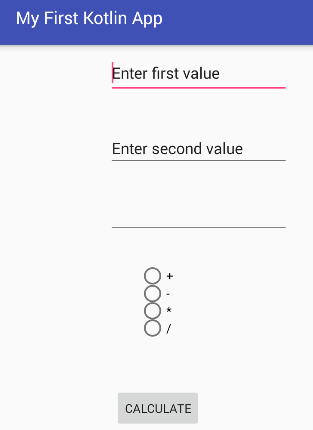You input texts for the first value and second value as follows: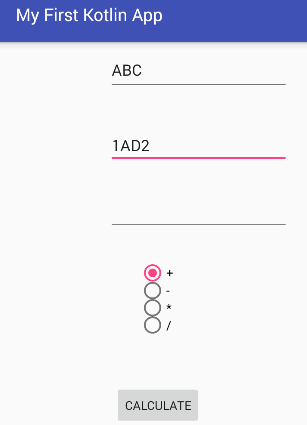Choose an operator and click the  `CALCULATE ` button, the result looks like this: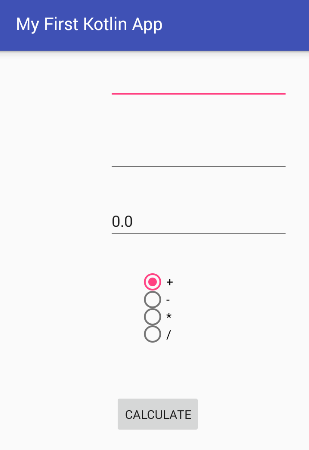## Conclusion

You can download my source here and I hope you have a great experience.